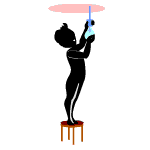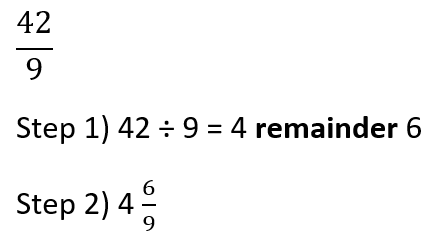# Math Worksheets Land

Math Worksheets For All Ages

# Math Worksheets Land

Math Worksheets For All Ages

Home > Math Topics > Fractions >

# Improper Fractions to Mixed Numbers Worksheets

I run into this skill every time I have a pizza party whether it is at school or at home. Everyone always likes a different type of pizza, so we always end up buying 4 or 5 different types of pizza. At the end of the party, we have a whole bunch of left-over slices. We always want to keep the pizza for later. I mean, whoever throws out pizza? To save space, we combine all the slices into as few boxes as possible. This is where we run into converting those fractions (pizza slices) into a mixed number (the whole value represents how many pizza boxes). We will work with this skill which is a relatively simple two step process. If you need a refresher on how to perform this operation check the lesson sheet below or scroll down for a live example. A selection of worksheets and lessons that teach students the steps required to convert a top-heavy fraction to a mixed number.

### Aligned Standard: 4.NF.B.3b

• Answer Keys - These are for all the unlocked materials above.

### Homework Sheets

Time to fatten up your fractions. We break it into two pretty simple steps for students.

• Homework 1 - Write the mixed number as an improper fraction.
• Homework 2 - Step #1: Find the product of the whole number and denominator.
• Homework 3 - Step #2: Find the sum of that number and the numerator.

### Practice Worksheets

Note the changes in format with these sheets. Numbers 1 and 3 go in one direction and number 2 goes in another.

### Math Skill Quizzes

Once again, note the transformation that each set takes on below.

### How Do You Convert an Improper Fraction to a Mixed Number?When studying fractions, students come across two types of fractions, including proper fractions and improper fractions. Proper fractions are the ones where the numerator is smaller than the denominator. The values of these fractions are within 0 and 1. Then there are improper fractions or top-heavy fractions, where the numerators are greater than the denominators. The value of these fractions is always greater than 1.

There is another way to write improper fractions, and that is what we call mixed numbers or mixed fractions. A mixed fraction is one where you write a whole number beside a proper fraction. It comprises of a quotient, divisor, and remainder. You can very easily convert an improper fraction into a mixed number.

Step 1: Division - Use the traditional division method to divide the numerator by the denominator. Identify the quotient, dividend, and the remainder. The number of times the denominator goes into the numerator will be the whole number value. The remainder will serve as the numerator of the fractional part and the denominator stays as is.

Step 2: Correctly Place the Number - The general form of a mixed number is; Quotient = Remainder / (Divisor)You place the quotient, remainder, and the divisor in their respective places. In these two simple steps, you can convert an improper fraction into a mixed number. Here is an example, convert the value 42/9 into a mixed number.

The numerator (42) serves as the dividend and the denominator (9) as the divisor. This result on 4 even divisions. 4 serves as the whole number. The remainder (6) serves as the numerator of the remaining fraction and the denominator (9) stays put.

Unlock all the answers, worksheets, homework, tests and more!
Save Tons of Time! Make My Life Easier Now

## Thanks and Don't Forget To Tell Your Friends!

I would appreciate everyone letting me know if you find any errors. I'm getting a little older these days and my eyes are going. Please contact me, to let me know. I'll fix it ASAP.